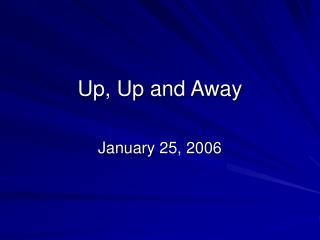DownloadDownload PresentationUp, Up and Away

# Up, Up and Away

Télécharger la présentation## Up, Up and Away

- - - - - - - - - - - - - - - - - - - - - - - - - - - E N D - - - - - - - - - - - - - - - - - - - - - - - - - - -
##### Presentation Transcript

1. Up, Up and Away January 25, 2006

2. Calendar • Today • Some problems on vectors • Introduction to some 2D issues • Friday, Monday • 2D Kinematics • Wednesday • Exam #1 – Through 2D Stuff finished on Monday • Friday • Going around in circles

3. Find the horizontal and vertical components of the d = 140 m displacement of a superhero who flies from the top of a tall building following the path shown in the Figure where = 30.0°. Superperson

4. The helicopter view in Figure P3.35 shows two people pulling on a stubborn mule. Let the magnitude of F2 = 64.0 N and the angle at which F1 pulls be = 65.0°. • Find the single force that is equivalent to the two forces shown. The forces are measured in units of newtons (symbolized N). • (b) Find the force that a third person would have to exert on the mule to make the resultant force equal to zero. • Use I,j notation

5. 2 Dimensional Motion • We will consider motion the the x-y plane. • Positions now have (x,y) coordinates so we need to use vectors. • That’s why we did that pointy stuff on Monday • There are two types of problems we need to consider • Throw or drop an object at an angle to the horizontal • Make something go around in a circle

6. Positions are VECTOR quantities

7. Velocities

8. Which way is the acceleration?

9. In 2D motion, lots of things happen at once! Velocity Position

10. An Example … What is its velocity here? It’s acceleration? How long did it take to get here? here? height (h) RANGE (R)

11. Let’s Just Do it!